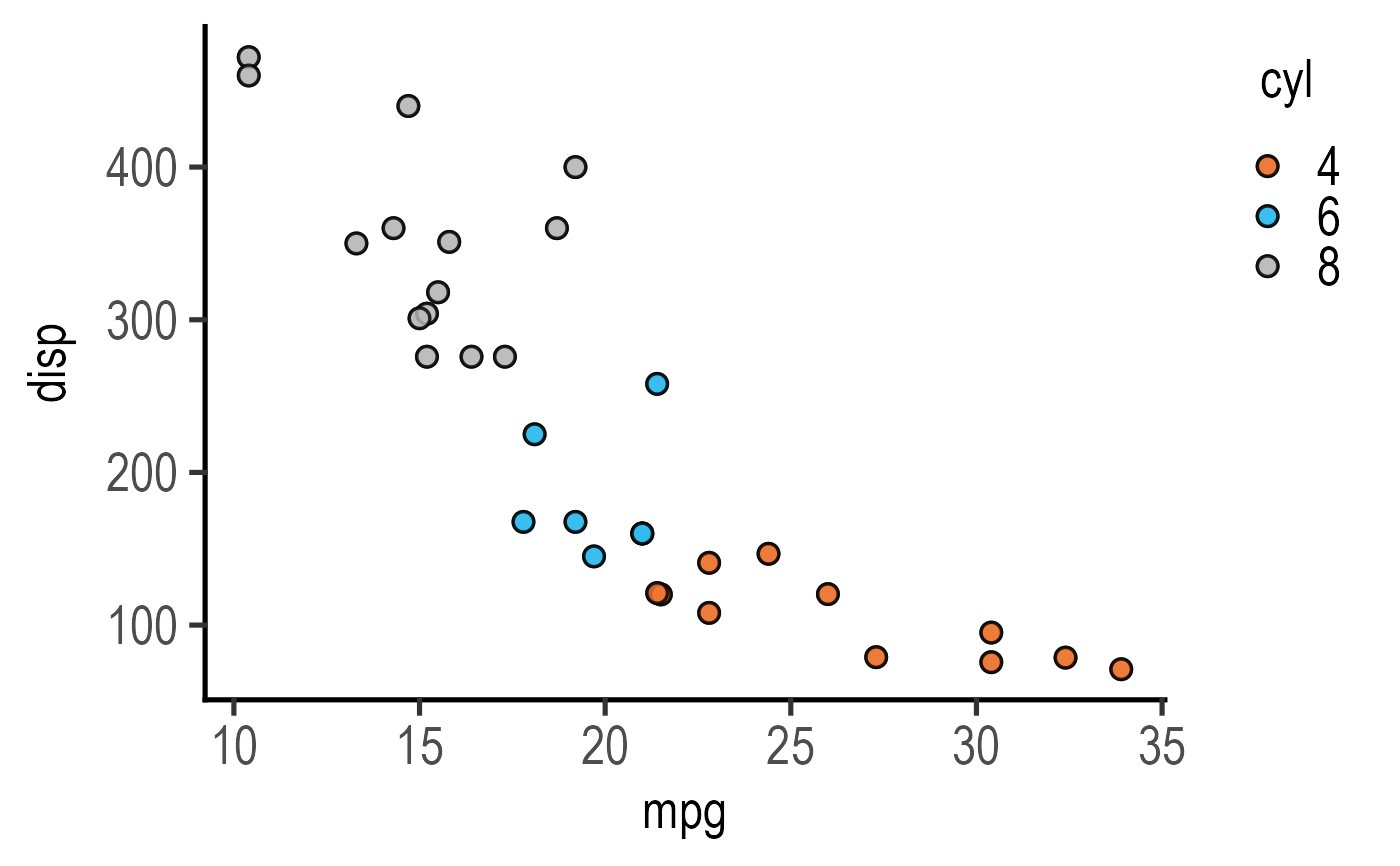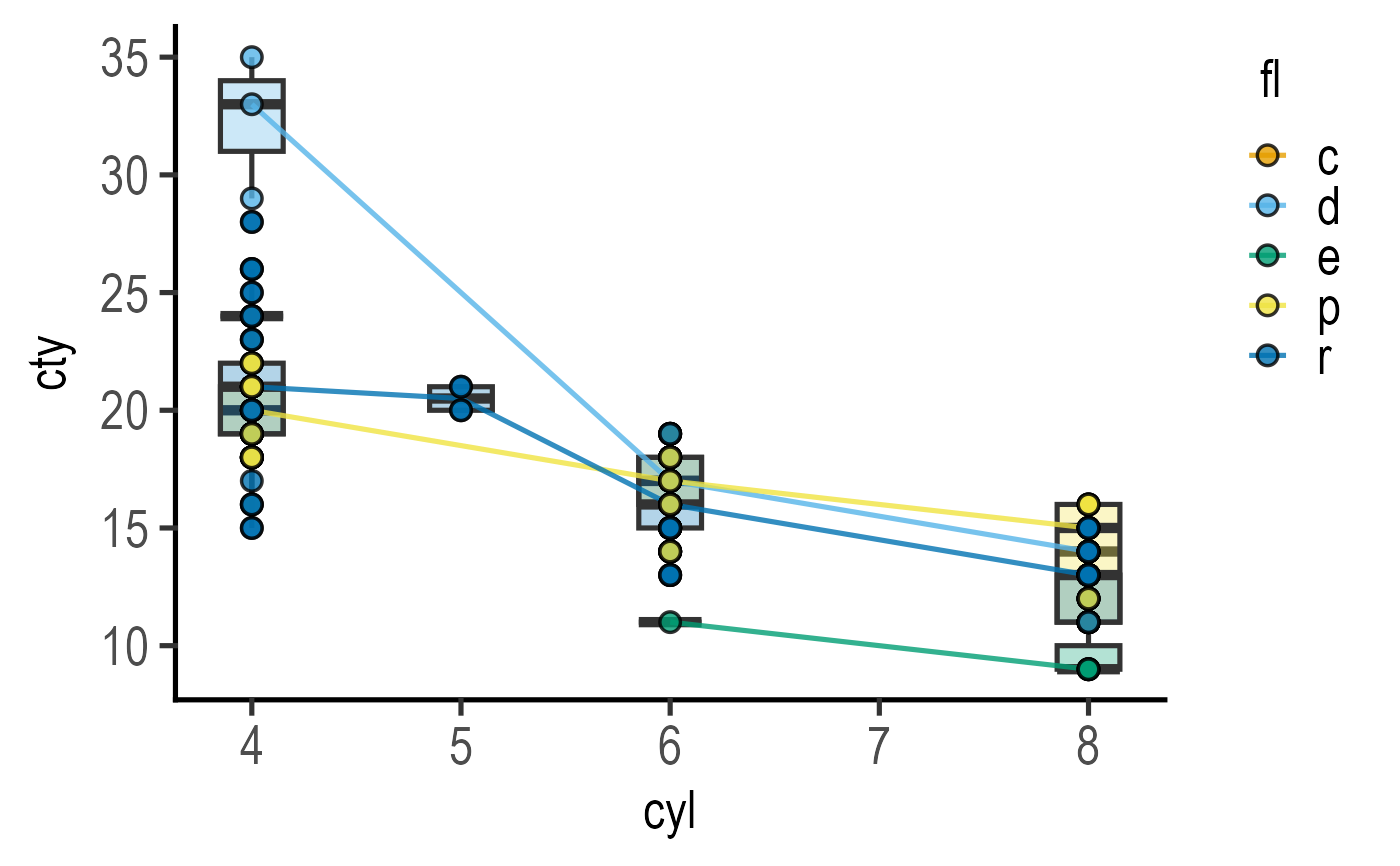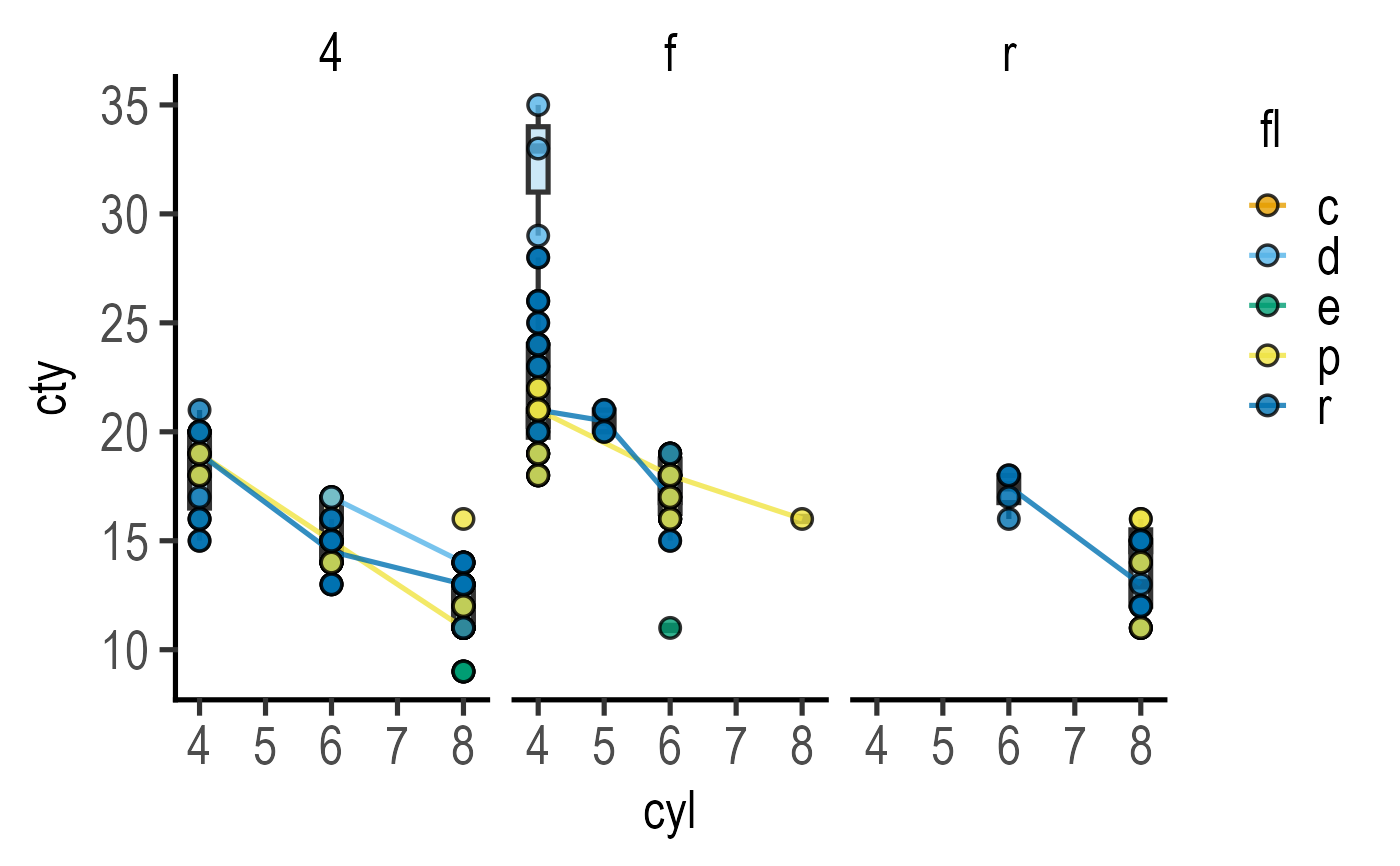This function takes a data table, quantitative X and Y variables along with a categorical grouping variable, and a and plots a graph with using geom_point. The categorical CatGroup variable is mapped to the fill aesthetic of symbols.

plot_xy_CatGroup(
data,
xcol,
ycol,
CatGroup,
facet,
Boxplot = FALSE,
symsize = 3,
s_alpha = 0.8,
TextXAngle = 0,
LogYTrans,
LogXTrans,
LogYBreaks = waiver(),
LogXBreaks = waiver(),
LogYLabels = waiver(),
LogXLabels = waiver(),
LogYLimits = NULL,
LogXLimits = NULL,
facet_scales = "fixed",
fontsize = 20,
bwid = 0.3,
b_alpha = 0.3,
l_alpha = 0.8,
symthick,
bthick,
ColPal = c("okabe_ito", "all_grafify", "bright", "contrast", "dark", "fishy", "kelly",
"light", "muted", "pale", "r4", "safe", "vibrant"),
ColSeq = TRUE,
ColRev = FALSE,
...
)

## Arguments

data

a data table object, e.g. data.frame or tibble.

xcol

name of the column with quantitative X variable.

ycol

name of the column with quantitative Y variable.

CatGroup

a categorical variable as grouping factor for colour of data points, should be a categorical variable for default colours to work. Will be converted to factor if your column is numeric

facet

add another variable from the data table to create faceted graphs using ggplot2facet_wrap.

Boxplot

logical TRUE/FALSE to plot box and whiskers plot (default = FALSE).

symsize

size of symbols used by geom_point. Default set to 3.

s_alpha

fractional opacity of symbols, default set to to 0.8 (i.e, 80% opacity).

TextXAngle

orientation of text on X-axis; default 0 degrees. Change to 45 or 90 to remove overlapping text.

LogYTrans

transform Y axis into "log10" or "log2"

LogXTrans

transform X axis into "log10" or "log2"

LogYBreaks

argument for ggplot2[scale_y_continuous] for Y axis breaks on log scales, default is waiver(), or provide a vector of desired breaks.

LogXBreaks

argument for ggplot2[scale_x_continuous] for Y axis breaks on log scales, default is waiver(), or provide a vector of desired breaks.

LogYLabels

argument for ggplot2[scale_y_continuous] for Y axis labels on log scales, default is waiver(), or provide a vector of desired labels.

LogXLabels

argument for ggplot2[scale_x_continuous] for Y axis labels on log scales, default is waiver(), or provide a vector of desired labels.

LogYLimits

a vector of length two specifying the range (minimum and maximum) of the Y axis.

LogXLimits

a vector of length two specifying the range (minimum and maximum) of the X axis.

facet_scales

whether or not to fix scales on X & Y axes for all graphs. Can be fixed (default), free, free_y or free_x (for Y and X axis one at a time, respectively).

fontsize

parameter of base_size of fonts in theme_classic, default set to size 20.

bwid

width of boxplot (default = 0.3).

b_alpha

fractional opacity of boxes, (default = 0.3).

l_alpha

fractional opacity of lines joining boxes, (default = 0.8).

symthick

size (in 'pt' units) of outline of symbol lines (stroke), default = fontsize/22.

bthick

size (in 'pt' units) of outline of boxes, whisker and joining lines (stroke), default = fontsize/22.

ColPal

grafify colour palette to apply, default "okabe_ito"; see graf_palettes for available palettes.

ColSeq

logical TRUE or FALSE. Default TRUE for sequential colours from chosen palette. Set to FALSE for distant colours, which will be applied using scale_fill_grafify2.

ColRev

whether to reverse order of colour within the selected palette, default F (FALSE); can be set to T (TRUE).

...

any additional arguments to pass on.

## Value

This function returns a ggplot2 object of class "gg" and "ggplot".

## Details

A box and whisker plot with lines joining the medians can be plotted with Boxplot = TRUE. If only box plot is needed without the line, set the opacity of the line to 0 (i.e., l_alpha = 0).

Colours can be changed using ColPal, ColRev or ColSeq arguments. Colours available can be seen quickly with plot_grafify_palette. ColPal can be one of the following: "okabe_ito", "dark", "light", "bright", "pale", "vibrant, "muted" or "contrast". ColRev (logical TRUE/FALSE) decides whether colours are chosen from first-to-last or last-to-first from within the chosen palette. ColSeq (logical TRUE/FALSE) decides whether colours are picked by respecting the order in the palette or the most distant ones using colorRampPalette.

This plot is related to plot_xy_NumGroup which requires a numeric grouping factor. When summary statistics (mean/median) are required, use plot_3d_scatterbar, plot_3d_scatterbox or plot_4d_scatterbox.

## Examples

#The grouping factor cyl  is automatically converted to categorical variable
plot_xy_CatGroup(data = mtcars,
xcol = mpg, ycol = disp, CatGroup = cyl,
ColPal = "vibrant", ColSeq = FALSE)#with boxplot
plot_xy_CatGroup(data = mpg,
xcol = cyl, ycol = cty,
CatGroup = fl, Boxplot = TRUE)